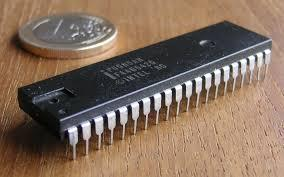20 Questions | Total Attempts: 184Settings.

• 1.
The number of hardware interrupts that the processor 8085 HAS
• A.

1

• B.

3

• C.

5

• D.

7

• 2.
The register that stores all the interrupt requests in it in order to serve them one by one on priority basis is
• A.

Interrupt Request Register

• B.

In-Service Register

• C.

Priority resolver

• D.

• 3.
Which statement is true for 8085 and 8086 clock speed?
• A.

8085 - 3 Mhz, 8086 - 10 Mhz

• B.

8085 - 3 Mhz , 8086 - 5,8 Mhz

• C.

None of the Options

• D.

8085 - 3 Mhz , 8086 - 5,8, 10 Mhz

• 4.
The interrupts have the following pin nos
• A.

6 to 10

• B.

31 to 35

• C.

6 to 12

• D.

5 to 9

• 5.
Which triggers in 8085 are level triggered?
• A.

RST 6.5, RST 5.5 , TRAP

• B.

RST 6.5, RST 7.5

• C.

RST 6.5 and RST 5.5

• D.

TRAP

• 6.
You need to set the interrupt masks so that RST5.5 is enabled, RST6.5 is masked, and RST7.5 is enabled. What is the content of the accumulator?
• A.

0A H

• B.

0B H

• C.

0C H

• D.

0D H

• 7.
8085 and 8086 have how many flags respectively?
• A.

5,8

• B.

5,7

• C.

5,9

• D.

5,6

• 8.
8086 supports pipelining because
• A.

It has EU and BIU separately

• B.

It supports MIN and MAX mode

• C.

BIU supports pre fetching

• D.

It is 16 bit microprocessor

• 9.
8086 is 16 a bit microprocessor
• A.

Hence supports word length of 16 bit

• B.

Because it has 16 bit data bus

• C.

Because it has word length of 8 and data bus of 16 bit

• D.

None of the Options

• 10.
8086 has how many vectored interrupts?
• A.

5

• B.

4

• C.

12

• D.

None of the Options

• 11.
How many hardware interrupts support masking by more than 1 way?
• A.

5

• B.

4

• C.

2

• D.

3

• 12.
EI and DI has instructiuon length of how many bytes?
• A.

1

• B.

2

• C.

3

• D.

None of the Options

• 13.
Why TRAP is edge and level sensitive?
• A.

Because to avoid trigeering by only level

• B.

To avoid false triggering because of noise

• C.

To avoid false triggering

• D.

None of the Options

• 14.
In how many ways RST 5.5, RST 6.5 and RST 7.5 can be disabled?
• A.

1

• B.

2

• C.

3

• D.

4

• 15.
Let RST 7.5 is to be masked (disabled), while RST 6.5 and RST 5.5 are to be unmasked (enabled), then the content of the bits of the SIM instruction will be like
• A.

0A H

• B.

0B H

• C.

0C H

• D.

0D H

• 16.
RIM instruction is exceuted , RST 6.5 is pending , then what is the content of the accumulator?
• A.

10 H

• B.

20 H

• C.

30 H

• D.

40 H

• 17.
What is the use of TRAP Interrupt?
• A.

• B.

IO Interfacing

• C.

DMA Request

• D.

Interrupt in Power Failure

• 18.
RST 7.5 is
• A.

• B.

• C.

• D.

Maskable , Hardwired, Vectored, Level Sensitive

• 19.
How many interrupts SIM and DI disable respectively?
• A.

3,4

• B.

0-3,4

• C.

2,4

• D.

3,3

• 20.
Which interrupt requires a separate programmable interrupt controller?
• A.

INTR

• B.

TRAP

• C.

RST 7.5

• D.

RST 6.5

Related TopicsBack to top